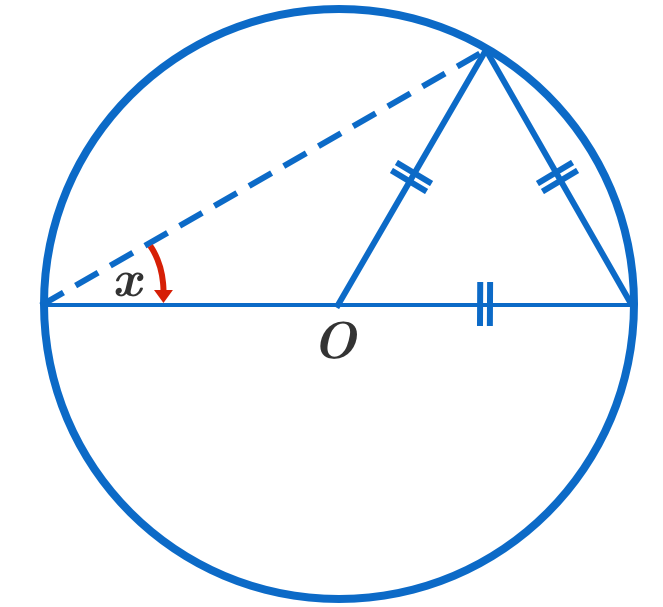# Is Thales' Theorem Necessary?

Geometry Level 1An equilateral triangle inscribed inside a circle such that two of its vertices touches the circumference of the circle and one its vertices touches the center of the circle, $O$. What is the measure of $x$ in degrees?

×